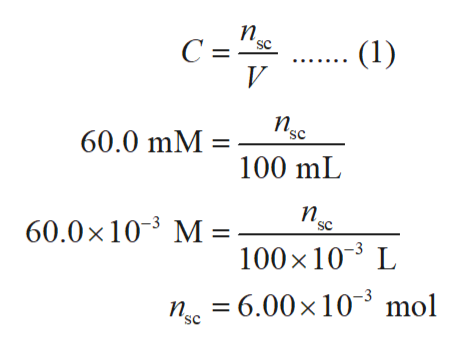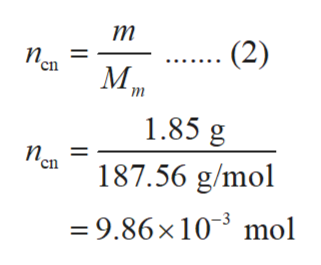# Hello, this is one of the problems that I cannot figure out on my homework. Thank you for your help. Suppose 1.85g of copper(II) nitrate is dissolved in 100.mL of a 60.0mM aqueous solution of sodium chromate.Calculate the final molarity of copper(II) cation in the solution. You can assume the volume of the solution doesn't change when the copper(II) nitrate is dissolved in it.Be sure your answer has the correct number of significant digits.

Question
977 views

Hello, this is one of the problems that I cannot figure out on my homework. Thank you for your help.

Suppose 1.85g of copper(II) nitrate is dissolved in 100.mL of a 60.0mM aqueous solution of sodium chromate.

Calculate the final molarity of copper(II) cation in the solution. You can assume the volume of the solution doesn't change when the copper(II) nitrate is dissolved in it.

check_circle

Step 1

The number of moles of sodium chromate (nsc) is calculated using equation (1) in which C is the concentration of sodium chromate and V is the volume of solution.help_outlineImage Transcriptioncloseп, ..... 1) C ="sc V n 60.0 mM 100 mL п. SC 60.0x 103 M 100x 10 L 6.00x 103 mol n SC fullscreen
Step 2

The number of moles of copper nitrate (ncn) is calculated using equation (2) in which m is the mass of solute and Mm is the molar mass of solute.help_outlineImage Transcriptioncloseт .. (2) п. en М, 1.85 g п. 187.56 g/mol cn 3 mol =9.86x 10 fullscreen
Step 3

The reaction between copper nitrate and...

### Want to see the full answer?

See Solution

#### Want to see this answer and more?

Solutions are written by subject experts who are available 24/7. Questions are typically answered within 1 hour.*

See Solution
*Response times may vary by subject and question.
Tagged in

### General Chemistry